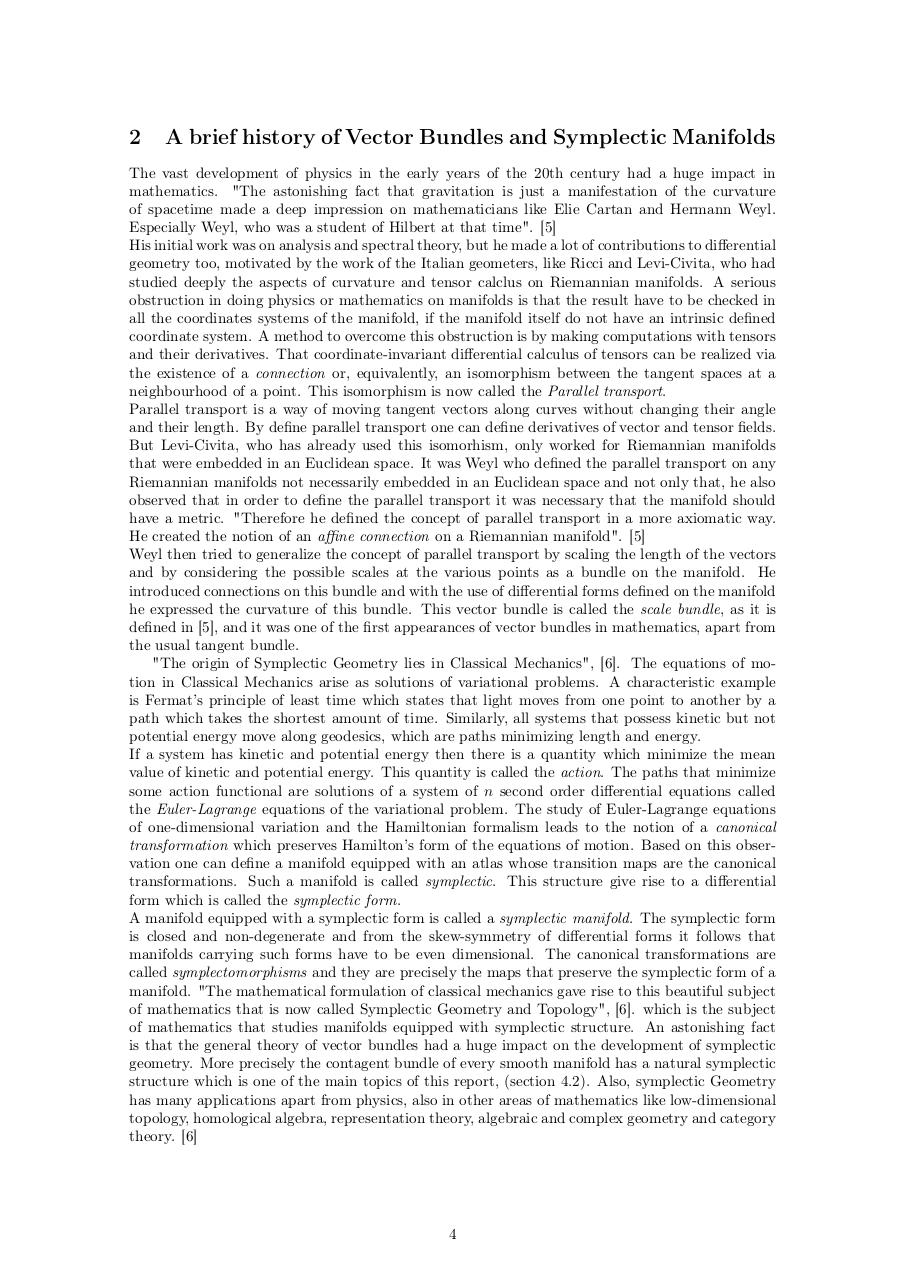# Functors in Differential Geometry.pdfPage 1 2 3 45614

#### Text preview

2

A brief history of Vector Bundles and Symplectic Manifolds

The vast development of physics in the early years of the 20th century had a huge impact in
mathematics. &quot;The astonishing fact that gravitation is just a manifestation of the curvature
of spacetime made a deep impression on mathematicians like Elie Cartan and Hermann Weyl.
Especially Weyl, who was a student of Hilbert at that time&quot;. 
His initial work was on analysis and spectral theory, but he made a lot of contributions to differential
geometry too, motivated by the work of the Italian geometers, like Ricci and Levi-Civita, who had
studied deeply the aspects of curvature and tensor calclus on Riemannian manifolds. A serious
obstruction in doing physics or mathematics on manifolds is that the result have to be checked in
all the coordinates systems of the manifold, if the manifold itself do not have an intrinsic defined
coordinate system. A method to overcome this obstruction is by making computations with tensors
and their derivatives. That coordinate-invariant differential calculus of tensors can be realized via
the existence of a connection or, equivalently, an isomorphism between the tangent spaces at a
neighbourhood of a point. This isomorphism is now called the Parallel transport.
Parallel transport is a way of moving tangent vectors along curves without changing their angle
and their length. By define parallel transport one can define derivatives of vector and tensor fields.
But Levi-Civita, who has already used this isomorhism, only worked for Riemannian manifolds
that were embedded in an Euclidean space. It was Weyl who defined the parallel transport on any
Riemannian manifolds not necessarily embedded in an Euclidean space and not only that, he also
observed that in order to define the parallel transport it was necessary that the manifold should
have a metric. &quot;Therefore he defined the concept of parallel transport in a more axiomatic way.
He created the notion of an affine connection on a Riemannian manifold&quot;. 
Weyl then tried to generalize the concept of parallel transport by scaling the length of the vectors
and by considering the possible scales at the various points as a bundle on the manifold. He
introduced connections on this bundle and with the use of differential forms defined on the manifold
he expressed the curvature of this bundle. This vector bundle is called the scale bundle, as it is
defined in , and it was one of the first appearances of vector bundles in mathematics, apart from
the usual tangent bundle.
&quot;The origin of Symplectic Geometry lies in Classical Mechanics&quot;, . The equations of motion in Classical Mechanics arise as solutions of variational problems. A characteristic example
is Fermat’s principle of least time which states that light moves from one point to another by a
path which takes the shortest amount of time. Similarly, all systems that possess kinetic but not
potential energy move along geodesics, which are paths minimizing length and energy.
If a system has kinetic and potential energy then there is a quantity which minimize the mean
value of kinetic and potential energy. This quantity is called the action. The paths that minimize
some action functional are solutions of a system of n second order differential equations called
the Euler-Lagrange equations of the variational problem. The study of Euler-Lagrange equations
of one-dimensional variation and the Hamiltonian formalism leads to the notion of a canonical
transformation which preserves Hamilton’s form of the equations of motion. Based on this observation one can define a manifold equipped with an atlas whose transition maps are the canonical
transformations. Such a manifold is called symplectic. This structure give rise to a differential
form which is called the symplectic form.
A manifold equipped with a symplectic form is called a symplectic manifold. The symplectic form
is closed and non-degenerate and from the skew-symmetry of differential forms it follows that
manifolds carrying such forms have to be even dimensional. The canonical transformations are
called symplectomorphisms and they are precisely the maps that preserve the symplectic form of a
manifold. &quot;The mathematical formulation of classical mechanics gave rise to this beautiful subject
of mathematics that is now called Symplectic Geometry and Topology&quot;, . which is the subject
of mathematics that studies manifolds equipped with symplectic structure. An astonishing fact
is that the general theory of vector bundles had a huge impact on the development of symplectic
geometry. More precisely the contagent bundle of every smooth manifold has a natural symplectic
structure which is one of the main topics of this report, (section 4.2). Also, symplectic Geometry
has many applications apart from physics, also in other areas of mathematics like low-dimensional
topology, homological algebra, representation theory, algebraic and complex geometry and category
theory. 

4